# Overview

In this in-class lab our goal is to project a population with 3 age-groups using matrix multiplication. We are trying to learn several things:

1. How matrix multiplication takes account of births and deaths in an age-structured pop

2. Constant age-specific rates –> a steady state (“stable” population)

3. “Stable” populations have constant exponential growth rates and age distributions

(Note: at the end of this lab is a section we will use next week when we look at the demographic transition with changing age-specific rates.)

# 0. Population projection as a set of transitions

Let’s draw on the board the transitions between

• young (aged 0-19)
• middle aged (aged 20-39)
• old (aged 40-59)

from the year 2000 to the year 2020.

We should now have a “connected graph”, showing us the transitions between states.

Let’s put the same information in an “Input-Output” table, with input-states on the columns, and output-states on the rows.

## | Young (in) | Middle (in) | Old (in) |

Young (out) | | | | Middle (out) | | | | Old (out) | | | |

• Which of these table entries could be positive?
• Which will be “structural” zeros?

# 1. Matrix equation for population projection

$\mathbf{A}\cdot \mathbf{P}\left(t\right)=\mathbf{P}\left(t+n\right)$

where * $n$$n$ is projection step length * $A$$A$ is the projection matrix * $P$$P$ is a column vector of population by age group

Writing out the matrices,

$\left(\begin{array}{ccc}{F}_{I}& {F}_{II}& {F}_{III}\\ {S}_{I}& 0& 0\\ 0& {S}_{II}& 0\end{array}\right)\left(\begin{array}{c}{P}_{1}\left(t\right)\\ {P}_{II}\left(t\right)\\ {P}_{III}\left(t\right)\end{array}\right)=\left(\begin{array}{c}{P}_{1}\left(t+n\right)\\ {P}_{II}\left(t+n\right)\\ {P}_{III}\left(t+n\right)\end{array}\right)$

Let’s multiply out on the board, and compare to our “connected graph” of the transitions.

Let’s make sure we understand that our matrix multiplication is doing what we want.

# 2. An example

Let’s take a specific example of a projection matrix.

$\mathbf{A}=\left(\begin{array}{ccc}.6& .8& 0\\ .9& 0& 0\\ 0& .7& 0\end{array}\right)$
Let’s start with a young’ish population with more young than old (the youngest age group is the upper-most element).

$\mathbf{P}\left(0\right)=\left(\begin{array}{c}50\\ 30\\ 20\end{array}\right)$
Let’s answer the following questions (first by hand, …, and then we can check with ‘R’)

A. What is population after one projection step, $P\left("1"\right)$$P("1")$?

B. What is ratio of total population size at “1” to time “0”?

C. What is the annual population growth rate from “0” to “1” if we assume a projection step is 20 years?

## A. 1 projection step

A = matrix(c(.6, .8, 0,
.9, 0, 0,
0, .7, 0), ncol = 3, byrow = T)
P0 = matrix(c(50,
30,
20), ncol = 1, byrow = T)
P1 = A %*% P0  # '%*%' means matrix multiplication
print(P1)
     [,1]
[1,]   54
[2,]   45
[3,]   21

## B. Ratio of population sizes

P0.total = sum(P0)
P1.total = sum(P1)
my.ratio = P1.total / P0.total
print(my.ratio)
 1.2

## C. Annual growth rate

R.0.to.1 = log(P1.total/P0.total)/20
print(R.0.to.1)
 0.009116078

## D. Age-structure

Did the age-structure (proportion in each age group) change when we projected ${P}_{0}$$P_0$ forward in time?

## let's use the notation "little p" for proportions
p0 = P0/sum(P0)
p1 = P1/sum(P1)
print(cbind(P0, p0, P1, p1))
     [,1] [,2] [,3]  [,4]
[1,]   50  0.5   54 0.450
[2,]   30  0.3   45 0.375
[3,]   20  0.2   21 0.175

Is there an age structure that we can project that will not change? Let’s try

${\mathbf{P}}^{\mathrm{\prime }}\left(0\right)=\left(\begin{array}{c}45.7\\ 34.3\\ 20\end{array}\right)$

P0.prime = c(45.7, 34.2, 20)
P1.prime = A %*% P0.prime  # '%*%' means matrix multiplication
P1.prime = as.vector(P1.prime)
p0.prime = P0.prime/sum(P0.prime)
p1.prime = P1.prime/sum(P1.prime)
out <- round(cbind(P0.prime, p0.prime, P1.prime,  p1.prime),3)
print(out)
     P0.prime p0.prime P1.prime p1.prime
[1,]     45.7    0.457    54.78    0.457
[2,]     34.2    0.342    41.13    0.343
[3,]     20.0    0.200    23.94    0.200

Do proportions change?

Let’s visualize

plot(p0.prime, type = "b", col = "blue", lwd = 2)
lines(p1.prime, lty = 2, col = "red", lwd = 4)
title("Proportions in each age group, before and after projection")
legend("topright", legend = c("p.prime(0)", "p.prime(1)"), col = c("blue", "red"), lwd = c(2, 4))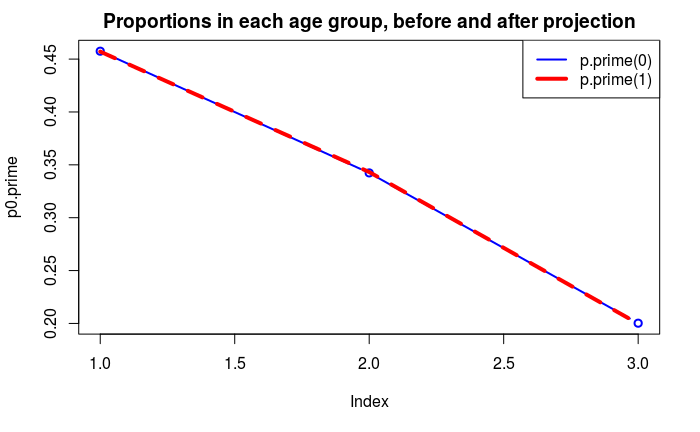# 3. Longer-term projection

Let’s say that demographic rates stay the same, and so the projection matrix stays the same. What happens in the long-term to * the rate of population growth? * the age structure?

We can use the computer to project a longer time, say 10 steps.

## approach 1: repeated multiplication
P1 = A %*% P0
P2 = A %*% P1
# etc .....
## approach 2: Iteration
P.mat = matrix(NA, nrow = 3, ncol = 10+1) # create an empty pop matrix
P.mat[,1] = P0 # initialize by putting starting population in first column
for (i in 1:10) # loop
{
P.mat[,i+1] = A %*% P.mat[,i] #result goes in i+1 column
}
## let's plot the results
matplot(1:11, t(P.mat), type = "o", main = "Age groups, regular scale")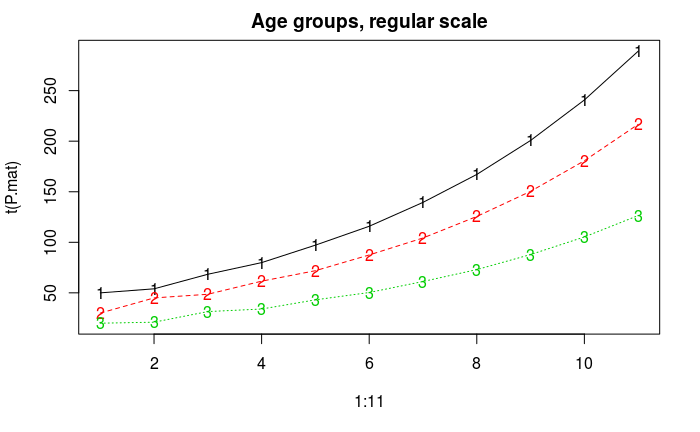Looks like exponential growth. We can check if we get straight lines in log-scale

matplot(1:11, t(P.mat), log = "y", type = "o", main = "Age groups, log scale")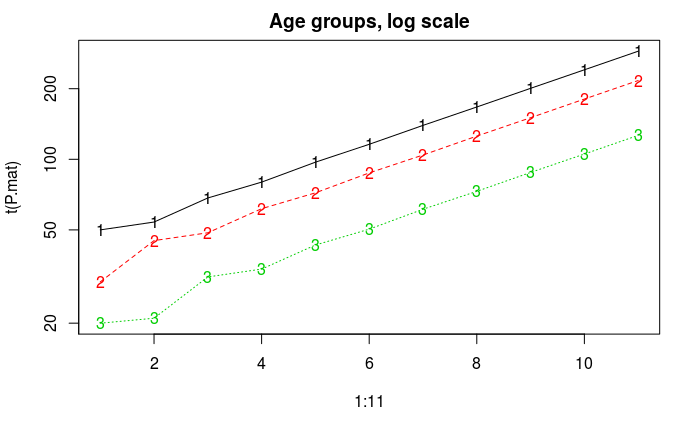## What is the “stable” growth rate?

Let’s plot the total population for each time period

P.total.vec = NULL
for (i in 1:11)
P.total.vec[i] = sum(P.mat[,i])
plot(1:11, P.total.vec)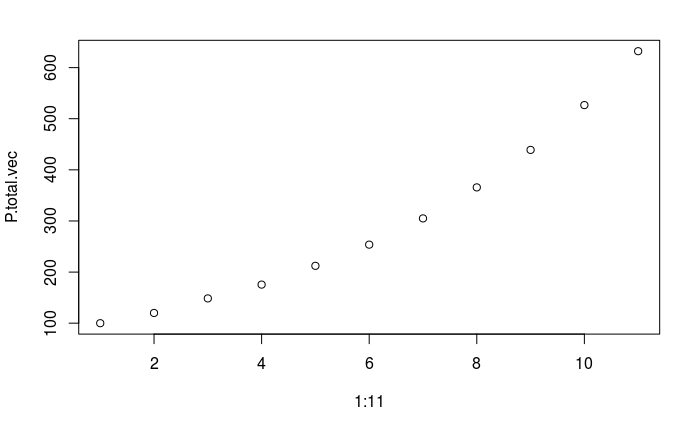Now, let’s calculate exponential growth rate and plot

R.vec = diff(log(P.total.vec))/ 20
plot(R.vec, type = "b")
abline(h = log(eigen(A)\$val)/20, col = "green")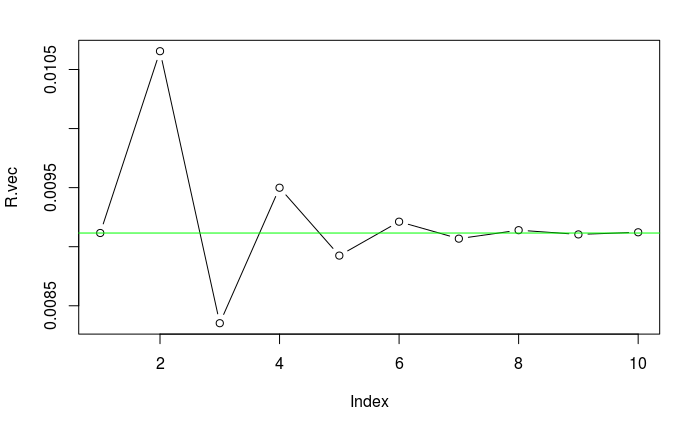and print out

print(round(R.vec, 3)) ## looks like long term growth rate is about .9 percent
  0.009 0.011 0.008 0.009 0.009 0.009 0.009 0.009 0.009 0.009

## What happens to the age stucture?

Let’s do an “animated” population pyramid

for (i in 1:10)
barplot(P.mat[,i], main = paste("step", i), names.arg = c("y", "m", "o"), horiz = T)